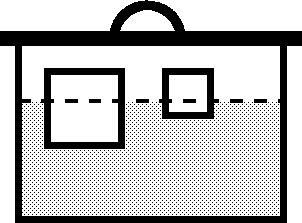시간 제한 메모리 제한 제출 정답 맞은 사람 정답 비율
1 초 128 MB 0 0 0 0.000%

## 문제

Some amount of water is poured into a barrel, then a number of cubes of different size and density are put into water. Finally, a lid is put onto the barrel and pushed down until it touches the edges of the barrel.Write a program to compute the resulting water level in the barrel.

It can be assumed that:

• the density of water is 1.0,
• the influence of air can be neglected,
• the cubes fit completely into the barrel,
• the cubes do not rotate and do not touch each other.

## 입력

The first line of the input contains three real numbers --- the bottom area of the barrel, S (0 < S ≤ 1000), the height of the barrel, H (0 < H ≤ 1000), and the volume of water, V (0 < V ≤ S∙H). The next line contains the number of cubes, N (0 < N ≤ 1000). It is followed by N lines, each containing two real numbers describing a cube --- the length of a side of the cube, L (0 < L ≤ 1000), and the density of the cube, D (0 < D ≤ 10).

## 출력

The first and only line of the output must contain one real number --- the resulting water level. The output must not differ from the correct value by more than 10-4.

## 예제 입력 1

100 10 500
1
1 0.5


## 예제 출력 1

5.0050